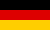Bri's worldelectronics, programming and more

deutschFirst you have to enter the desired output voltage. The button Start is used to start the calculation.

The results will be shown in the result table below.

Name Description Value
V_out Output voltage V
E series preferred values

Result

R2 output voltage
calculated
single resistor
two resistors parallel
two resistors series

R2 output voltage Vref = 1.25V (typ.) output voltage Vref = 1.2V (min.) output voltage Vref = 1.3V (max.)